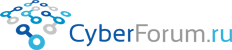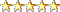Наши страницы
8Observer8
 Войти Регистрация Восстановить пароль
 Тесты Блоги Социальные группы Поиск Сообщения за день Все разделы прочитаны# Specifications for Calculator using Jasmine and JavaScript 5

Запись от 8Observer8 размещена 23.02.2019 в 15:14
Обновил(-а) 8Observer8 23.02.2019 в 15:29

Specifications:
• "Add(a, b)" method must to sum positive numbers. Specification name: Add_SumPositiveNumbers_ReturnsSum
• "Sub(a, b)" method must to subtract positive numbers. Specification name: Sub_SubtractPositiveNumbers_ReturnsSub

Source Code: https://github.com/8Observer8/calculator-jasmine-es5

Instruction:
• Create the "calculator-jasmine-es5" folder
• Search in the Internet: cdn jasmine
• Create the "SpecRunner.html file in the "calculator-jasmine-es5" folder and add links on files:
PHP/HTML
 ```1 2 3 4 5 6 7 8 9 10 11 12 13 14 15 16 17 18 19 20 21 22 23 24 ``` ```                   Jasmine Spec Runner v3.3.0                                             ```
Note. Files "calculator_tests.js" was not created yet but we added links on them above.
• Create the "src_client" folder. Create the "calculator.js" file the the "src_client" folder
• Create the "src_specs" folder. Create the "calculator_tests.js" file the the "src_specs" folder
• Add our specs described above to the "calculator_tests.js" file:

calculator_tests.js

Javascript
 ```1 2 3 4 5 6 7 8 9 10 11 12 13 14 15 16 17 18 19 20 21 22 23 24 25 26 27 28 29 30 31 32 ``` ```describe("Calculator", function() {     it("Add_SumPositiveNumbers_ReturnsSum", function()     {         // Arrange         var calculator = new Calculator();         var a = 5;         var b = 2;         var expectedSum = 7;           // Act         var actualSum = calculator.Add(a, b);           // Assert         expect(actualSum).toEqual(expectedSum);     });       it("Sub_SubtractPositiveNumbers_ReturnsSub", function()     {         // Arrange         var calculator = new Calculator();         var a = 5;         var b = 2;         var expectedSub = 3;           // Act         var actualSub = calculator.Sub(a, b);           // Assert         expect(actualSub).toEqual(expectedSub);     }); });```
• Open the "SpecRunner.html" in a browser to run tests. You will see errors in the browser because we did not implement the methods: Add(a, b) and Sub(a, b)
• Let's implement these methods and open the "SpecRunner.html" in the browser again to run tests. You will see that the tests are passed.

calculator.js

Javascript
 ```1 2 3 4 5 6 7 8 9 10 11 12 13 14 ``` ```var Calculator = function() {   };   Calculator.prototype.Add = function(a, b) {     return a + b; };   Calculator.prototype.Sub = function(a, b) {     return a - b; };```Размещено в Без категории
Просмотров 124 Комментарии 0
Всего комментариев 0
Комментарии
 КиберФорум - форум программистов, компьютерный форум, программирование Реклама - Обратная связь
 Powered by vBulletin® Version 3.8.9Copyright ©2000 - 2019, vBulletin Solutions, Inc.